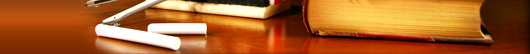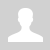Welcome Guest | RSSWelcome to science clubHome | Sign Up | Log In
 Site search
 Indian Heritage
 Social bookmarks
Home » 2014 » October » 29 » Forces between masses
9:17 AM
Forces between masses

# Forces between masses

Gravity is arguably the first force that people really learn about. People don't really think of it as learning about gravity because it is such a big part of our everyday lives. Babies learning to crawl or walk are struggling against gravity, games involving jumping, climbing or balls all give people a sense of the effect of gravity. The saying “everything that goes up, must come down” is all about gravity. Rain falls from the sky because of gravity and so much more. We all know that these things happen but we don't often stop to ask what is gravity, what causes it and how can we describe it more accurately than “everything that goes up, must come down”?

All of the examples mentioned involve objects with mass falling to the ground, they are being attracted to the Earth. Gravity is the name we give to the force that arises between objects because of their mass. It is always an attractive force. It is a non-contact force, therefore it acts at a distance. The Earth is responsible for a gravitational force on the moon which keeps it in its orbit around the Earth and the gravitational force the moon exerts on the Earth is the dominant cause of ocean tides.

Sir Isaac Newton was the first scientist to precisely define the gravitational force, and to show that it could explain both falling bodies and astronomical motions. The gravitational force is relatively simple. It is always attractive, and it depends only on the masses involved and the distance between them. Stated in modern language, Newton’s universal law of gravitation states that every particle in the universe attracts every other particle with a force along a line joining them. The force is directly proportional to the product of their masses and inversely proportional to the square of the distance between them.

## Newton's law of universal gravitation

Definition 1: Newton's law of universal gravitation

Every point mass attracts every other point mass by a force directed along the line connecting the two. This force is proportional to the product of the masses and inversely proportional to the square of the distance between them.

The magnitude of the attractive gravitational force between the two point masses, F is given by:where:is in newtons (N),is the gravitational constant 6,67 × 10−11 N·m2·kg−2is the mass of the first point mass in kilograms (kg),is the mass of the second point mass in kilograms (kg) andis the distance between the two point masses in metres (m). For any large objects (not point masses) we use the distance from the centre of the object(s) to do the calculation. This is very important when dealing with very large objects like planets. The distance from the centre of the planet and from the surface of the planet differ by a large amount. Remember that this is a force of attraction and should be described by a vector. We use Newton's law of universal gravitation to determine the magnitude of the force and then analyse the problem to determine the direction.

For example, consider a man of mass 80 kg standing 10 m from a woman with a mass of 65 kg. The attractive gravitational force between them would be:If the man and woman move to 1 m apart, then the force is:As you can see, these forces are very small.

Now consider the gravitational force between the Earth and the Moon. The mass of the Earth is 5,98 × 1024 kg, the mass of the Moon is 7,35 × 1022 kg and the Earth and Moon are 3,8 × 108 m apart. The gravitational force between the Earth and Moon is:From this example you can see that the force is very large.

These two examples demonstrate that the greater the masses, the greater the force between them. Thefactor tells us that the distance between the two bodies plays a role as well. The closer two bodies are, the stronger the gravitational force between them is. We feel the gravitational attraction of the Earth most at the surface since that is the closest we can get to it, but if we were in outer-space, we would barely feel the effect of the Earth's gravity!

Remember thatwhich means that every object on Earth feels the same gravitational acceleration! That means whether you drop a pen or a book (from the same height), they will both take the same length of time to hit the ground... in fact they will be head to head for the entire fall if you drop them at the same time. We can show this easily by using Newton's second law and the equation for the gravitational force. The force between the Earth (which has the mass) and an object of massis

and the acceleration of an object of mass(in terms of the force acting on it) is

So we equate them and we find thatSince it doesn't depend on the mass of the object,, the acceleration on a body (due to the Earth's gravity) does not depend on the mass of the body. Thus all objects experience the same gravitational acceleration. The force on different bodies will be different but the acceleration will be the same. Due to the fact that this acceleration caused by gravity is the same on all objects we label it differently, instead of usingwe usewhich we call the gravitational acceleration and it has a magnitude of approximately 9,8 m·s−2.

The fact that gravitational acceleration is independent of the mass of the object holds for any planet, not just Earth, but each planet will have a different magnitude of gravitational acceleration.

Views: 756 | Added by: scienceclub | Rating: 0.0/0CountryVisitorsscienceclub.ucoz.com This Website Visits